# Average numbers if not blank in Excel

In this article, we will learn how to get the Average numbers if not blank in Excel.

Problem?

For Instance, We have a large list of data and we need to find the Average of the price or amount given some criteria. Criteria is getting average only where cells are not blank. Criteria can be applied over any column of the data.

How to solve the Problem.

Now we will make a formula out of the function. Here we are given the data and we needed to find the AVERAGE of the numbers ignoring blank cells in Excel

Generic formula:

= AVERAGEIFS ( Average_range , range, "<>")
• Average_range : range of values where average needs to be evaluated
• range : range where criteria is applied.
• "<>" : criteria, ignores blank cells.

Example:

All of these might be confusing to understand. So, let's test this formula via running it on the example shown below.

Here we have given data(A1:D15) of received orders from different regions with given quantity & Price value. We need to find the AVERAGE of price where quantity is not blank.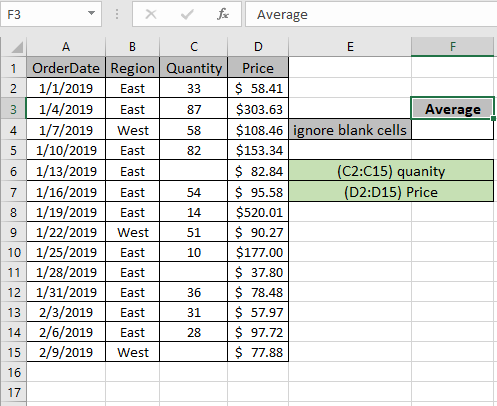The above condition or criteria needs to be matched. The AVERAGEIFS function can help us extract the average having not blank cells.

Use the Formula:

= AVERAGEIFS ( Price , quantity , "<>" )

Quantity : named range used for C2:C15 array

Price : named range used for D2:D15 array

"<>" : criteria

Explanation:

1. AVERAGEIFS function gets the average of range where all conditions satisfy.
2. Here the condition applies on quantity range i.e. average of price where quantity is not blank.
3. "<>" matches values where cells are not blank.

Here Named ranges given as (C2:C15) quantity and (D2:D15) Price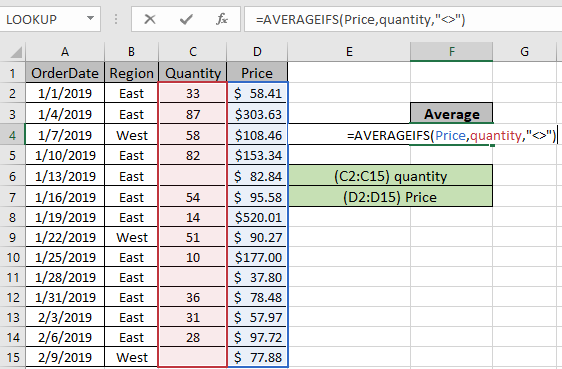The formula used in cell will be shown like the above snapshot. Press Enter to get the Price average if quantity is not blank.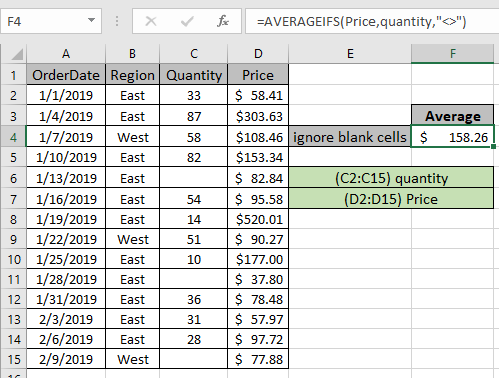As you can see in the above snapshot the AVERAGE of Prices where quantity is not blank comes out to be 158.26. You can check the result by applying filter on the data and filter non blank quantity values as shown below.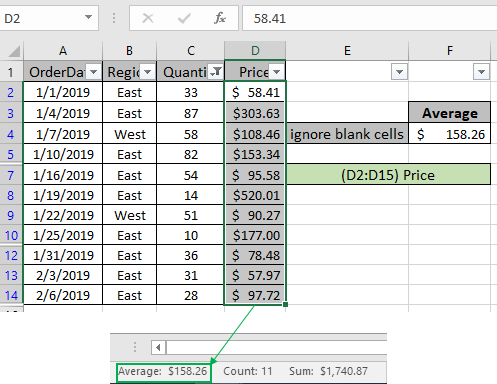The Average results will be seen in the bottom right of the excel worksheet along with count and Sum.

Example 2:
Here we have given data(A1:D15) of received orders from different regions with given quantity & Price value. We need to find the AVERAGE of price where quantity is not blank and region is East.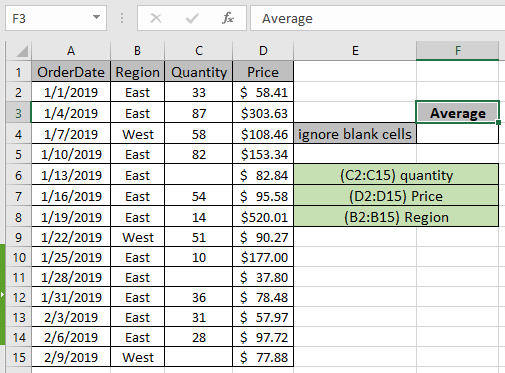This will include multiple criteria in the formula. The AVERAGEIFS function can help us extract the average having different criteria.
Use the Formula:

= AVERAGEIFS ( Price , quantity , "<>" , Region , "East" ) )

Region : named range used for B2:B15 array

Quantity : named range used for C2:C15 array

Price : named range used for D2:D15 array

"<>" : criteria

Explanation:

1. AVERAGEIFS function gets the average of range where all conditions satisfy.
2. Here the condition applies on quantity range i.e. average of price where quantity is not blank.
3. "<>" matches values where cells are not blank.
4. Region is the second criteria range where condition is Region array must match the value "East"

Here Named ranges given as (C2:C15) quantity , (D2:D15) Price & (B2:B15) Region.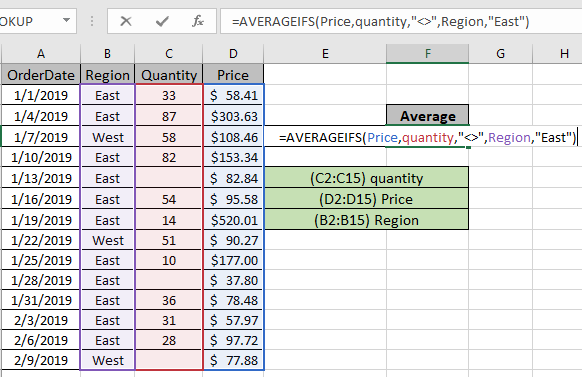The formula used in cell will be shown like the above snapshot. Press Enter to get the Price average if quantity is not blank and Region is East.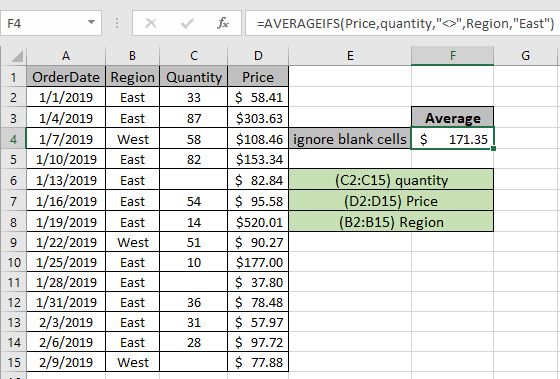As you can see in the above snapshot the AVERAGE of Prices where quantity is not blank comes out to be 171.35. You can check the result by applying filter on the data and filter non blank quantity values as shown below.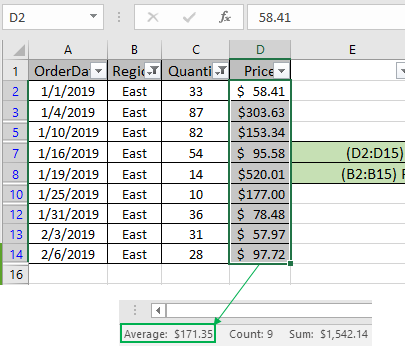The Average results will be seen in the bottom right of the excel worksheet along with count and Sum. This proves the formula works fine.

Here are some observations about the formula usage below.

Notes:

1.  The function returns #VALUE! error if the argument to the function is non-numeric.
2. The function returns #VALUE! error if no value matches the given criteria.
3.  Criteria operatorThe formula accepts such as equals to (=), less than (<) , less than or equals to (<=) , greater than or equals to (>=) , greater than (>) & not equals to (<>).

In this way, we learnt how to use the AVERAGEIFS function in Excel to get a return from the non-blank cells. You can use these functions in Excel 2016, 2013 and 2010. Find more articles on Mathematical formulation with condition here. If you have any issue regarding this article or any unresolved query, please comment in the comment box below. We will assist you.

Related Articles

How to use the AVERAGEIFS function in excel

How to use the DAVERAGE Function in Excel

How To Highlight Cells Above and Below Average Value

Ignore zero in the Average of numbers

Calculate Weighted Average

Average Difference between lists

Validation of text entries

Create drop down list in excel with colour

Popular Articles

Edit a dropdown list

Absolute reference in Excel

If with conditional formatting

If with wildcards

Vlookup by date

Join first and last name in excel

Count cells which match either A or B

Convert Inches To Feet and Inches in Excel 2016

50 Excel Shortcut to Increase Your Productivity

Terms and Conditions of use

The applications/code on this site are distributed as is and without warranties or liability. In no event shall the owner of the copyrights, or the authors of the applications/code be liable for any loss of profit, any problems or any damage resulting from the use or evaluation of the applications/code.